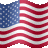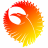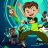# Momentum Oscillator with Volatility Squeeze for ThinkorSwim#### BenTen

Staff
VIP
Warehouse
One script but two indicators. A momentum oscillator with volatility squeeze created by Mobius.Here are his notes:

• Long Signal: When Momentum is ascending with value at or above 0 and green Point appears at zero line indicating the Volatility squeeze is over and volatility is expanding.
• Short Signal: When Momentum is descending with value above 0 and first yellow point appears a volatility compression has begun that will likely resolve to a lower value.
• Neutral: If Momentum is below 0 and Squeeze indicates the direction is indeterminate.
• Labels: Labels have been added for Squeeze Signals and Divergence Signals. Alter Momentum line to Weighted Scatterplot Loess line. More predictive

### thinkScript Code

Rich (BB code):
``````# Momentum Oscillator with Volatility Squeeze
# Mobius
# V01.06.2013

declare lower;

input MDetrend =  8; #hint MDetrend: Look-back period for Momentum oscillator
input fastEMA  = 20; #hint fastEMA: Exponential MA period for the Momentum oscillator
input slowEMA  =  5; #hint slowEMA: Exponential MA slowing period for the Momo oscillator
input AverageType = {default SMA, EMA, LRL};
input price = close; #hint price: value used in all calculations
input displace = 0;  #hint displace: displaced period for squeeze calculations
input length = 20;   #hint length: periods or length for the squeeze calculations
input Num_Dev_Dn = -2.0; #hint Num_Dev_Dn: Multiplier for the lower BB Band
input Num_Dev_Up = 2.0;  #hint Num_Dev_Up: Multiplier for the upper BB Band
input BLength = 20;      #hint BLength: periods or length for the bandwidth/squeeze calcs
input SLength = 20;      #hint SLength" periods or length for the squeeze calcs

script BB {
input price = close;
input displace = 0;
input length = 20;
input Numb_Dev_Dn = -2.0;
input Numb_Dev_Up = 2.0;
def StDev = StDev(price, length);
plot Mid = Inertia(price, length);
plot Up = Mid + (Numb_Dev_Up * StDev);
plot DN = Mid + (Numb_Dev_Dn * StDev);
}

def upperBand;
def lowerBand;
def midLine;

switch (AverageType) {
case SMA:
upperBand = reference BollingerBands(price, displace, length, Num_Dev_Dn, Num_Dev_Up).UpperBand;
lowerBand = reference BollingerBands(price, displace, length, Num_Dev_Dn, Num_Dev_Up).LowerBand;
midLine = reference BollingerBands(price, displace, length, Num_Dev_Dn, Num_Dev_Up).MidLine;
case EMA:
upperBand = reference BollingerBands(price, displace, length, Num_Dev_Dn, Num_Dev_Up, averageType = AverageType.EXPONENTIAL).UpperBand;
lowerBand = reference BollingerBands(price, displace, length, Num_Dev_Dn, Num_Dev_Up, averageType = AverageType.EXPONENTIAL).LowerBand;
midLine = reference BollingerBands(price, displace, length, Num_Dev_Dn, Num_Dev_Up, averageType = AverageType.EXPONENTIAL).MidLine;
case LRL:
upperBand = BB(price, displace, length, Num_Dev_Dn, Num_Dev_Up).Up;
lowerBand = BB(price, displace, length, Num_Dev_Dn, Num_Dev_Up).Dn;
midLine = BB(price, displace, length, Num_Dev_Dn, Num_Dev_Up).Mid;
}

def w = (upperBand - lowerBand) / midLine * 100;
def b = Highest(w, BLength);
def s = Lowest(w, SLength);

plot SqEnter = if w <= s
then 0
else Double.NaN;
SqEnter.SetPaintingStrategy(PaintingStrategy.POINTS);
SqEnter.SetLineWeight(4);
SqEnter.SetDefaultColor(Color.YELLOW);
plot SqExit = if w <= s and w > s
then 0
else Double.NaN;
SqExit.SetPaintingStrategy(PaintingStrategy.POINTS);
SqExit.SetLineWeight(4);
SqExit.SetDefaultColor(Color.GREEN);

# Momentum
plot M = ExpAverage(price - price[MDetrend], fastEMA);
M.SetPaintingStrategy(PaintingStrategy.HISTOGRAM);
M.SetLineWeight(5);
M.AssignValueColor(if M < 0 and M > M
then Color.LIGHT_GREEN
else if M > 0 and M > M
then Color.GREEN
else if M > 0 and M < M
then Color.BLUE
else Color.RED);
plot SlowM = ExpAverage(M, slowEMA);
SlowM.SetPaintingStrategy(PaintingStrategy.HISTOGRAM);
SlowM.SetLineWeight(5);
SlowM.AssignValueColor(if SlowM < 0 and SlowM > SlowM
then Color.LIGHT_GREEN
else if SlowM > 0 and SlowM > SlowM
then Color.GREEN
else if SlowM > 0 and SlowM < SlowM
then Color.BLUE
else Color.RED);

plot zero = if IsNaN(close)
then Double.NaN
else if IsNaN(SqEnter) or IsNaN(SqExit) then 0 else Double.NaN;
zero.SetPaintingStrategy(PaintingStrategy.POINTS);
zero.SetLineWeight(3);
zero.SetDefaultColor(Color.BLUE);

# Divergence
def mean = Inertia(HL2, length);
def lowestLow = if low > mean
then Double.NaN
else if low crosses below mean
then low
else if low < mean and
low < low and
low < lowestLow
then low
else lowestLow;
def lowestM = if low > mean
then Double.NaN
else if M crosses below 0
then M
else if M < 0 and
M < M and
M < lowestM
then M
else lowestM;
def Ldivergence = if low <= lowestLow and
M > lowestM
then 0
else Double.NaN;

def highestHigh = if high < mean
then Double.NaN
else if high crosses above mean
then high
else if high > mean and
high > high and
high > highestHigh
then high
else highestHigh;
def HighestM = if high < mean
then Double.NaN
else if M crosses above 0
then M
else if M > 0 and
M > M and
M > HighestM
then M
else HighestM;

def Hdivergence = if high <= highestHigh and
M < HighestM
then 0
else Double.NaN;

AddLabel(SqEnter == 0, "In Squeeze" , if M >= 0 and M > M
then Color.GREEN
else if M > 0 and M < M
then Color.RED
else Color.YELLOW);
AddLabel(SqExit == 0, "In Squeeze" , if M >= 0 and M > M
then Color.GREEN
else if M > 0 and M < M
then Color.RED
else Color.YELLOW);
AddLabel(Ldivergence == 0, "Divergent Low", Color.WHITE);
AddLabel(Hdivergence == 0, "Divergent High", Color.WHITE);

# End Code``````Indicators 0Indicators 2Indicators 0Indicators 83